# Low-dropout linear regulators double as voltage-supervisor circuits

## ON Semiconductor NCP500

William Lepkowski

EDN

Many low-dropout voltage regulators include an enable-input pin that can also serve as an inexpensive alternative to a voltage-supervisor IC. Although the enable pin normally serves as a means of shutting down the regulator's output to save power, a few discrete components ensure that the regulator's output will turn on and off at appropriate input voltages. Thus, you can use the circuit as a voltage supervisor or as a controlled-characteristic linear-voltage regulator.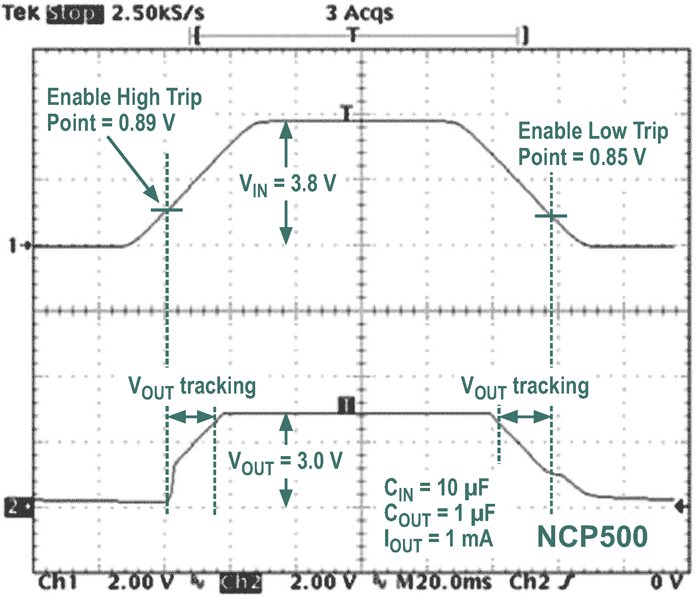Figure 1. Connecting a low-dropout regulator’s enable pin directly to the unregulated voltage input forces the output voltage to track the input voltage during the regulator’s turn-on and turn-off intervals.

A typical low-dropout regulator's internal enable circuit comprises a voltage comparator that determines whether the voltage at the enable pin is either larger or smaller than an internal reference voltage, VREF. Although you can create a low-dropout voltage supervisor by directly connecting the enable pin to the unregulated input voltage, this circuit's turn-on and turn-off voltages equal the reference voltage, which typically falls below the minimum operating voltage that most ICs powered by the regulator's output require.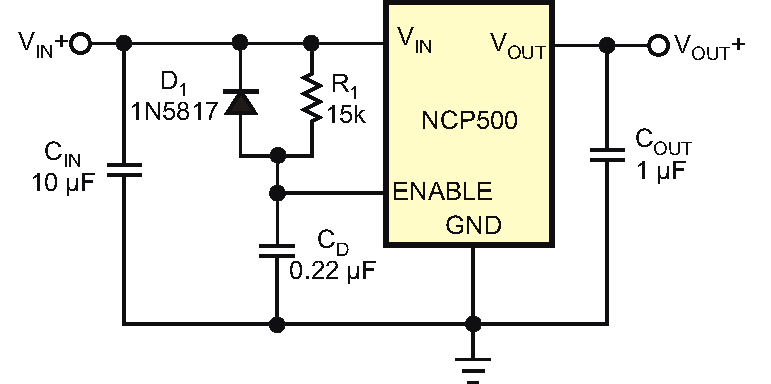Figure 2. An alternative to directly connecting the regulator’s input and enable pins, this “conventional” modification uses a resistor and a capacitor to delay the regulator’s turn-on time. The diode eliminates the powerdown delay interval.

In addition, directly connecting the enable pin to the unregulated input doesn't provide a turn-on delay to ensure that the input voltage has reached a value higher than the low-dropout regulator's dropout voltage. The directly connected circuit has unsatisfactory power-up and power-down characteristics (Figure 1). As a first-order improvement, you can enhance the circuit's performance by adding R1, CIN, and D1 to provide a start-up delay for the voltage regulator's enable pin (Figure 2). Unfortunately, the external delay network improves the output's rising-edge characteristic, but its falling edge continues to track the input voltage (Figure 3).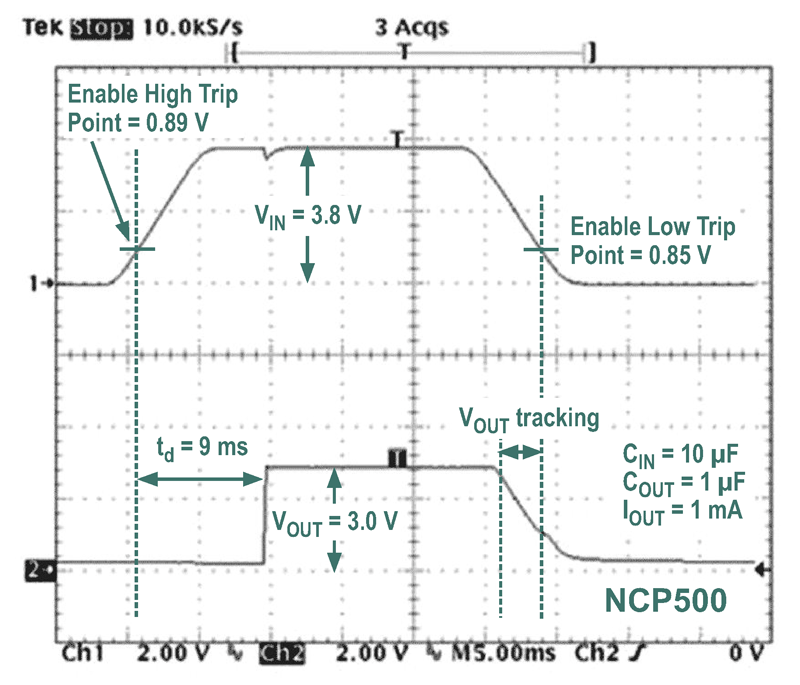Figure 3. The added components in Figure 2 eliminate the problem of rising-edge output-voltage tracking. However, the falling-edge output voltage still tracks the input voltage.

You can solve the circuit's shutdown problem by replacing the single resistor with a voltage-divider network (Figure 4). Resistor R2 raises the switching threshold of the regulator's enable pin and "tricks" the enable comparator into turning on at a higher voltage. The regulator's output then exhibits an adequate start-up delay and cleanly switches on and off (Figure 5).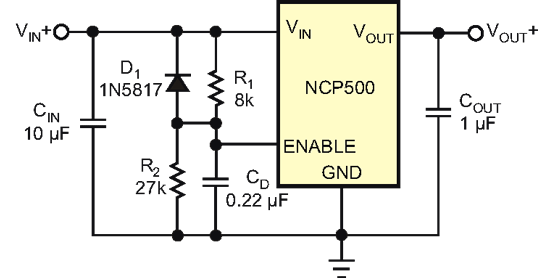Figure 4. Resistor R2 increases the enable pin’s switching threshold voltage.

You can use Equation 1 to calculate the values of resistors R1 and R2 to alter the enable pin's threshold voltage in the circuit in Figure 4.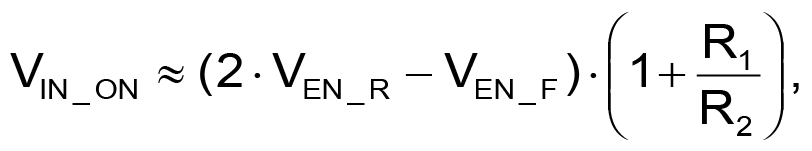(1)

where VIN_ON is the user-defined turn-on voltage, VEN_R is the enable pin's rising-edge trip-point voltage, and VEN_F is the enable pin's falling-edge trip-point voltage. For example VIN_ON = 4 V, VEN_R = 0.89 V, and VEN_F = 0.85 V. To prevent the regulated output voltage from tracking the input, set the minimum value of VIN_ON to VOUT + VDRP, where VDRP is the dropout voltage.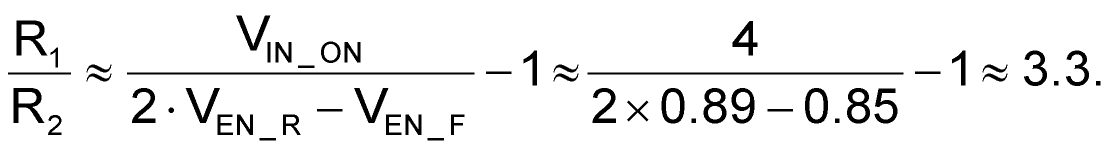(2)

If you select a value of 8 kΩ for R2, then R1=3.3×R2, or approximately 27 kΩ.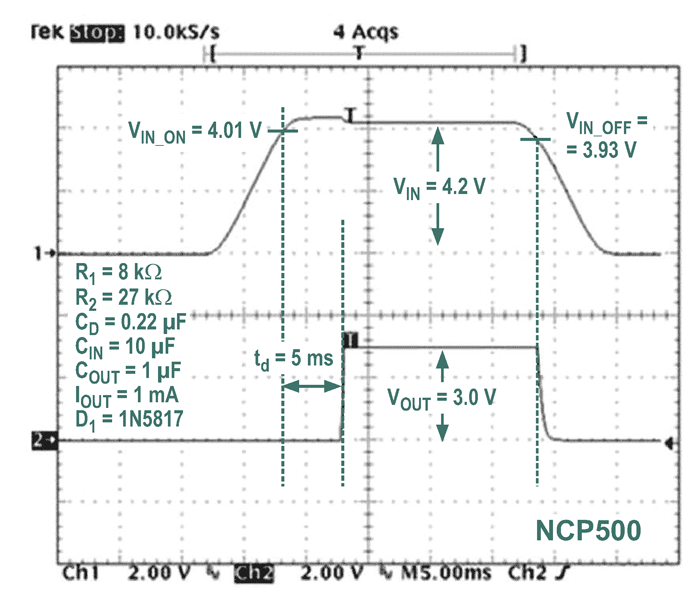Figure 5. The addition of R2 in Figure 3 solves the falling edge problem, and shutdown occurs immediately after the input voltage drops too low. The regulator’s output switches on only after sufficient voltage is present at its input.

Equation 1 calculates only approximate values for the voltage-divider resistors, which may vary slightly depending on the voltage regulator's characteristics. If the resistors' values are too low, the regulated output tracks the input, a problem that you can easily solve by increasing the value of R1. Also, R1 and CD determine the regulator's turn-on delay time, and CD's capacitance should ideally be 0.01 to 0.47 µF. Too large a value increases the discharge time and reduces the circuit's effectiveness as a voltage supervisor.

EDN